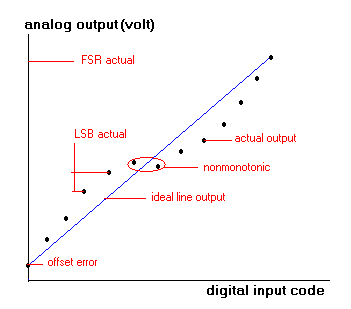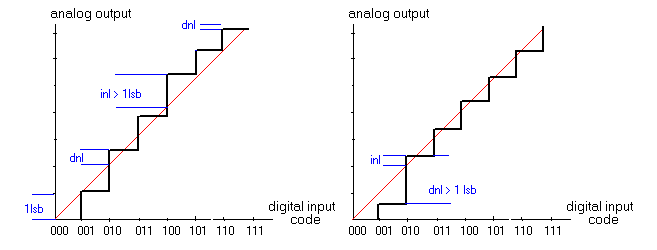HITEQUEST Apr 2002 DAC parameters

 This article is a brief memo about major DAC parameters.Fig.1   Resolution - normally given in bits.         Resolution indicates the smallest increment of its output,         corresponding to a 1 LSB input code change. For example for 10 bit DAC, 2 power 10 = 1024 codes,         so the resolution is 1/1024 of the output range. Full scale range (FSR) - maximum output signal for the DAC,specified as current or voltage (ma or V). Can be negative ,positive or both. Offset error   - difference between an ideal and actual DAC output when  zero digital code applied to the input.   Gain error  -  the difference between an ideal and actual output when full scale digital code applied to the input.      Strongly depends on VREF stability. Differential Nonlinearity - (see the drawing below)  is measured   whith a ramp code applied to the input of DAC.                             The step between every pair of the adjacent codes should not exceed 1 LSB.                             1LSB is calculated from gain and offset measurements:                                1 LSB = FSR/N-1          Differential Nonlinearity represents the error from 1 LSB for every step,                             where N=(2 power of bit number) .     Integral Nonlinearity - shows how the output differs from an ideal line. It is also measured in LSB. Usually 1LSB is an exeptable value for nonlinearity.    Fig.2 and Fig.3  show the difference between Integral and Differential Nonlinearity.    Offset and Gain are absolute measurements,DNL and INL are referenced to the    zero and full scale outputs of the DAC.   SNR - signal to noise ratio. Measured with digital code representing sine wave applied to the input. Fundamental    and harmonic components of the sine wave are filtered out.Any remaining signal at the output of the DAC    is considered as a noise.    SNR is a ratio of the full scale sine wave output to noise level.    Some companies specify SINAD parameter. SINAD - is a signal to noise and    distortion ratio. It is similar to SNR, but the harmonic signal components are not removed. Specified in dB. THD - total harmonic distortion  - measured with  digital code representing sinewave,applied to the DAC input continuously.    The output is analyzed in the frequency domain to find harmonic components related    to the fundamental output signal.Specified in dB.   IM - intermodulation distortion - non harmonic product terms that appear in the output signal due to nonlinearity    of the DAC. Measured with summed 2 sinusoid tone applied to the input.    The output is tested on  harmonic components,appearing due to    modulation affect on non linear characteristics within DAC.    The harmonic components are (F1+F2), (F1-F2) , (2F1+/-F2), (F1+/-2F2) ...   Max conversion rate - it is actually max input signal frequency the DAC can handle.The worst case is when    input signal changes from zero to max,and the output should reach the max level and settle. Settling time -  the time required for the output to reach the final value and remain within +-1 LSB    after overshoot. PSRR  - power supply rejection ratio .The output signal should remain within limits while power supply      voltage changes from Min to Max.Fig.2                                                                 Fig.3 Fig.2  shows the case when DNL  is within limits (<1 lsb) and INL is above 1lsb;   Fig.3 - the opposite case. If continuously increasing code from 0000 to 11111111 applied to the DAC input,the output should represent a rising staircase with equal steps. If staircase is smoothed,  a perfectly straight line should result. The INL defines the overall straightness of this line,  whereas the DNL describes differences in amplitude between adjacent steps in the staircase waveform.  Which of these two parameters is more important depends on the application.  In the imaging application, it may be necessary to distinguish between slightly different color densities  in adjacent areas of an image. Here DNL is more important .  But in an application in which a widely varying parameter like speed must be continuously monitored,  INL is usually more important. DNL must be less than 1lsb to garantee DAC's monotonic behavior (Fig.1). A Monotonic DAC  has an output that changes in the same direction (or remains constant) for each increase  in the input code. The quality of monotonicy is important if DAC is used in feedback loop. When a non monotonic device is used in a feedback loop, the loop can get stuck and DAC will toggle forever between 2 input codes. HITEQUEST Apr 2003